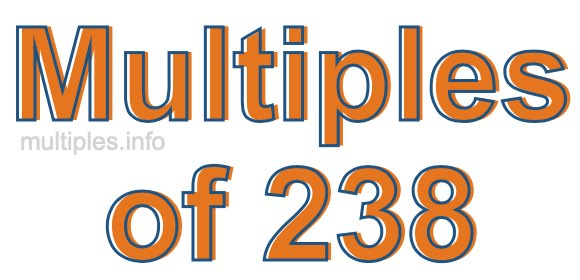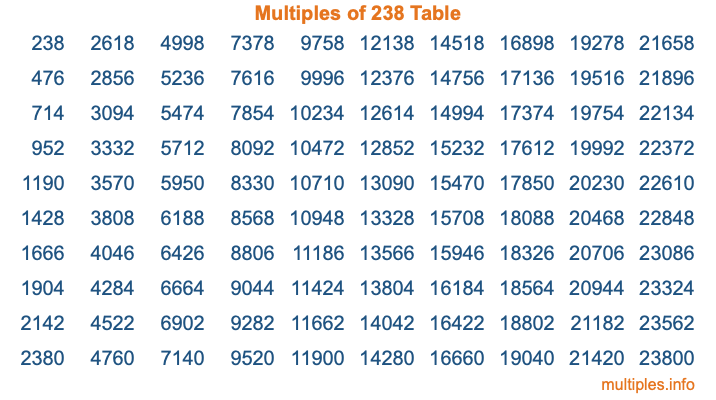Multiples of 238Welcome to the Multiples of 238 page. Here we will first teach you everything you will ever need to know about the multiples of 238, and then give you a study guide summary of everything we taught you to make sure you remember it all. Use this page to look up facts and learn information about the multiples of 238. This page will make you a multiples of two hundred thirty-eight expert!

Definition of Multiples of 238
Multiples of 238 are all the numbers that when divided by 238 equal an integer. Each of the multiples of 238 are called a multiple. A multiple of 238 is created by multiplying 238 by an integer.

Therefore, to create a list of multiples of 238, you start with 1 multiplied by 238, then 2 multiplied by 238, then 3 multiplied by 238, and so on for as long as you want. Thus, the list of the first five multiples of 238 is 238, 476, 714, 952, and 1190. To see a larger list of multiples of 238, see the printable image of Multiples of 238 further down on this page. We also have a category where you can choose any nth multiple of 238.

Multiples of 238 Checker
The Multiples of 238 Checker below checks to see if any number of your choice is a multiple of 238. In other words, it checks to see if there is any number (integer) that when multiplied by 238 will equal your number. To do that, we divide your number by 238. If the the quotient is an integer, then your number is a multiple of 238.

Is  a multiple of 238?

Least Common Multiple of 238 and ...
A Least Common Multiple (LCM) is the lowest multiple that two or more numbers have in common. This is also called the smallest common multiple or lowest common multiple and is useful to know when you are adding our subtracting fractions. Enter one or more numbers below (238 is already entered) to find the LCM.

Check out our LCM Calculator if you need more details about the Least Common Multiple or if you need the LCM for different numbers for adding and subtraction fractions.

nth Multiple of 238
As we stated above, 238 is the first multiple of 238, 476 is the second multiple of 238, 714 is the third multiple of 238, and so on. Enter a number below to find the nth multiple of 238.

th multiple of 238

Multiples of 238 vs Factors of 238
238 is a multiple of 238 and a factor of 238, but that is where the similarities end. All postive multiples of 238 are 238 or greater than 238. All positive factors of 238 are 238 or less than 238.

Below is the beginning list of multiples of 238 and the factors of 238 so you can compare:

Multiples of 238: 238, 476, 714, 952, 1190, etc.

Factors of 238: 1, 2, 7, 14, 17, 34, 119, 238

As you can see, the multiples of 238 are all the numbers that you can divide by 238 to get a whole number. The factors of 238, on the other hand, are all the whole numbers that you can multiply by another whole number to get 238.

It's also interesting to note that if a number (x) is a factor of 238, then 238 will also be a multiple of that number (x).

Multiples of 238 vs Divisors of 238
The divisors of 238 are all the integers that 238 can be divided by evenly. Below is a list of the divisors of 238.

Divisors of 238: 1, 2, 7, 14, 17, 34, 119, 238

The interesting thing to note here is that if you take any multiple of 238 and divide it by a divisor of 238, you will see that the quotient is an integer.

Multiples of 238 Table
Below is an image of the first 100 multiples of 238 in a table. The table is in chronological order, column by column. The first column has the first ten multiples of 238, the second column has the next ten multiples of 238, and so on.The Multiples of 238 Table is also referred to as the 238 Times Table or Times Table of 238. You are welcome to print out our table for your studies.

Negative Multiples of 238
Although not often discussed or needed in math, it is worth mentioning that you can make a list of negative multiples of 238 by multiplying 238 by -1, then by -2, then by -3, and so on, to get the following list of negative multiples of 238:

-238, -476, -714, -952, -1190, etc.

Multiples of 238 Summary
Below is a summary of important Multiples of 238 facts that we have discussed on this page. To retain the knowledge on this page, we recommend that you read through the summary and explain to yourself or a study partner why they hold true.

There are an infinite number of multiples of 238.

A multiple of 238 divided by 238 will equal a whole number.

238 divided by a factor of 238 equals a divisor of 238.

The nth multiple of 238 is n times 238.

The largest factor of 238 is equal to the first positive multiple of 238.

238 is a multiple of every factor of 238.

238 is a multiple of 238.

A multiple of 238 divided by a divisor of 238 equals an integer.

238 divided by a divisor of 238 equals a factor of 238.

Any integer times 238 will equal a multiple of 238.

Multiples of a Number
Here you can get the multiples of another number, all with the same attention to detail as we did for multiples of 238 on this page.

Multiples of
Multiples of 239
Did you find our page about multiples of two hundred thirty-eight educational? Do you want more knowledge? Check out the multiples of the next number on our list!

Copyright  |   Privacy Policy  |   Disclaimer  |   Contact